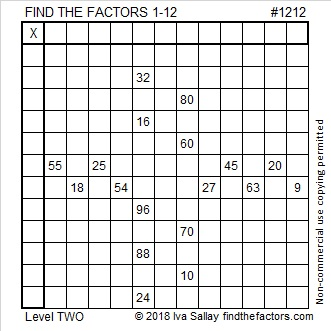# 1212 and Level 2

I know you will find at least some of today’s puzzle to be easy, and maybe even all of it. Why not give it a try? You might just have fun solving it, too!Print the puzzles or type the solution in this excel file: 12 factors 1211-1220

When we learn about the number 1212 we get to think about twelve a lot. 1212 has twelve factors and, of course, one of them is 12.

• 1212 is a composite number.
• Prime factorization: 1212 = 2 × 2 × 3 × 101, which can be written 1212 = 2² × 3 × 101
• The exponents in the prime factorization are 2, 1, and 1. Adding one to each and multiplying we get (2 + 1)(1 + 1)(1 + 1) = 3 × 2 × 2 = 12. Therefore 1212 has exactly 12 factors.
• Factors of 1212: 1, 2, 3, 4, 6, 12, 101, 202, 303, 404, 606, 1212
• Factor pairs: 1212 = 1 × 1212, 2 × 606, 3 × 404, 4 × 303, 6 × 202, or 12 × 101
• Taking the factor pair with the largest square number factor, we get √1212 = (√4)(√303) = 2√303 ≈ 34.813791212 is the sum of the fourteen prime numbers from 59 to 113.
It is also the sum of the twelve prime numbers from 73 to 131.
Do you know what all those prime numbers are?

1212 is the hypotenuse of a Pythagorean triple:
240-1188-1212 which is 12 times (20-99-101)